# A stake is being pulled out of the ground by means of two ropes as shown, Knowing that the tension in one rope is Problem 1.- A stake is being pulled out of the ground by means of two ropes as shown, Knowing that the tension in one rope is 120 N, determine by trigonometry the magnitude and direction of the force P so that the resultant is a vertical force of 160 N

Question-AnswerCategory: Engineering MechanicsA stake is being pulled out of the ground by means of two ropes as shown, Knowing that the tension in one rope is Problem 1.- A stake is being pulled out of the ground by means of two ropes as shown, Knowing that the tension in one rope is 120 N, determine by trigonometry the magnitude and direction of the force P so that the resultant is a vertical force of 160 N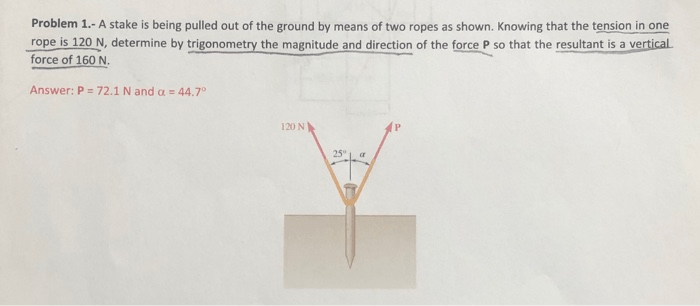Problem 1.- A stake is being pulled out of the ground by means of two ropes as shown, Knowing that the tension in one rope is 120 N, determine by trigonometry the magnitude and direction of the force P so that the resultant is a vertical force of 160 N

Given data :

• Force by rope 1 ,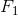= 120 N at angle of 25° from vertical axis in anticlockwise direction
• Force by rope 2,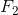= P Newton at angle of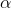° from vertical axis in clockwise direction
• Resultant force , R = 160 N in verticle upward directionNow, as shown in figure we can get two component ofand, in vertical and horizontal direction.
So, from figure we say that,
Resultant force in horizontal direction,But it is given that, resultant force must be in vertical dorection only. So,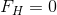So,So,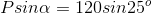So,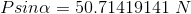________(1)
Same way, Resultant force in vertical direction,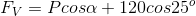We have given resultant force in vertical direction i.e. 160 N so,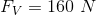So,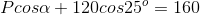So,_______(2)
Now taking square of equation (1) and equation (2) and then adding them ,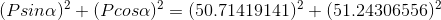So,Now,,
So,So,Now, from equation (1),So,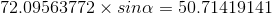So,So,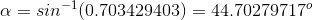So, Force by rope 2 is 72.1 N and angleis equal to 44.7° .